# BITSAT Logical Reasoning Test - 1

## 10 Questions MCQ Test BITSAT Mock Tests Series & Past Year Papers | BITSAT Logical Reasoning Test - 1

Description
Attempt BITSAT Logical Reasoning Test - 1 | 10 questions in 10 minutes | Mock test for JEE preparation | Free important questions MCQ to study BITSAT Mock Tests Series & Past Year Papers for JEE Exam | Download free PDF with solutions
QUESTION: 1

### Choose the pair in which the words are differently related.

Solution:

In all other pairs, second is a part of the first.

QUESTION: 2

### Complete the analogous pair. Command : Order : : Confusion : ?

Solution:

The word in each pair are synonyms.

QUESTION: 3

### Find the missing character.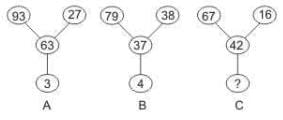Solution:

In A, 93 – (63 + 27) = 3
In B, 79 – (37 + 38) = 4

By sensing the pattern:
In C, 67 – (42 + 16) = 9

QUESTION: 4

Find out the term in the number series which is wrong.
1, 2, 4, 8, 16, 32, 64, 96

Solution:

Each term of the series is obtained by multiplying the preceding term by 2.

So, 96 is wrong and must be replaced by (64 * 2)  i.e. 128

QUESTION: 5

Find out the term in the number series which is wrong.
89, 78, 86, 80, 85, 82, 83

Solution:

Take even place numbers: 78, 80, 82

78 - 80 = 2
80 - 82 = 2

Take odd place numbers: 89, 86, 85, 83

89 - 86 = 3
86 - 85 = 1
85 - 83 = 2

If we change the number 87 instead of 86 we will get the difference are 2, 2, 2.
So, the odd term is 86.

QUESTION: 6

Group the following figures into three classes on the basis of identical properties.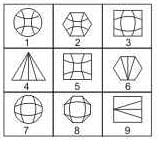Solution:

Groups formed:
1, 2, 5
3, 7, 8
4, 6, 9

QUESTION: 7

Select the correct mirror-image of the Figure (X) from amongst the given alternatives.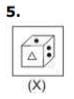Solution:

Because in mirror image right becomes left and left becomes right.

QUESTION: 8

Select the correct mirror-image of the Figure (X) from amongst the given alternatives.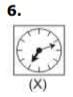Solution:

Because in mirror image right becomes left and left becomes right.

QUESTION: 9

Each of the following questions consists of five Problem figures followed by five Answer figures marked A, B, C, D, and E . Select a figure from the Answer Figures which will continue the same series as given in the Problem figures.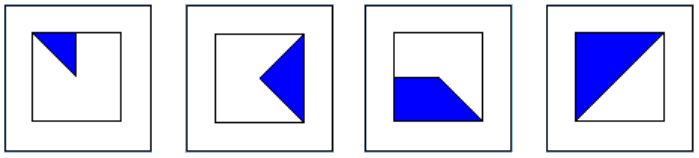Solution:

Each box increments by one blue segment (one eight of the square). So there are 4 segments in box four (half the square is filled).
So there must be 5 blue segments (5/8th of the square) in the answer which leaves us with A or D.
But the diagonal edge of the first segment is also rotating clockwise by 90% each time, so it should be aligned NW in the answer.

QUESTION: 10

Each of the following questions consists of five Problem figures followed by five Answer figures marked A, B, C, D, and E . Select a figure from the Answer Figures which will continue the same series as given in the Problem figures.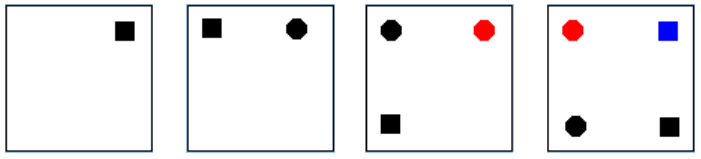Solution:

Items are introduced in this order: black square, black dot, red dot, blue square, green dot. New items are always introduced into the top right corner.Use Code STAYHOME200 and get INR 200 additional OFF Use Coupon Code